## Selina Concise Mathematics Class 9 ICSE Solutions Triangles [Congruency in Triangles]

APlusTopper.com provides step by step solutions for Selina Concise Mathematics Class 9 ICSE Solutions Chapter 9 Triangles [Congruency in Triangles]. You can download the Selina Concise Mathematics ICSE Solutions for Class 9 with Free PDF download option. Selina Publishers Concise Mathematics for Class 9 ICSE Solutions all questions are solved and explained by expert mathematic teachers as per ICSE board guidelines.

Selina ICSE Solutions for Class 9 Maths Chapter 9 Triangles [Congruency in Triangles]

Exercise 9(A)

Solution 1: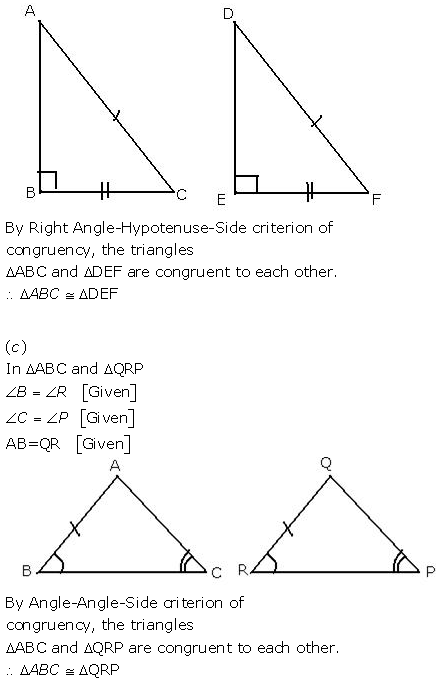Solution 2: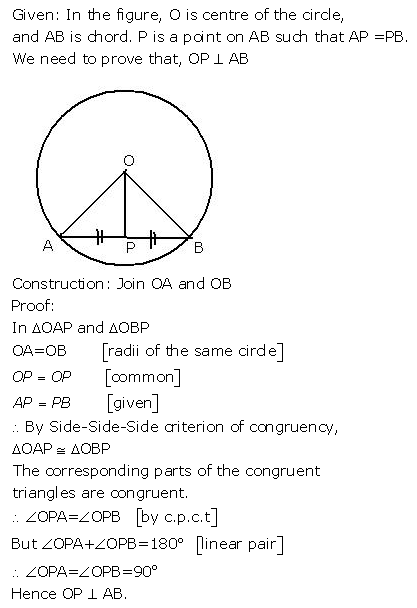Solution 3:Solution 4: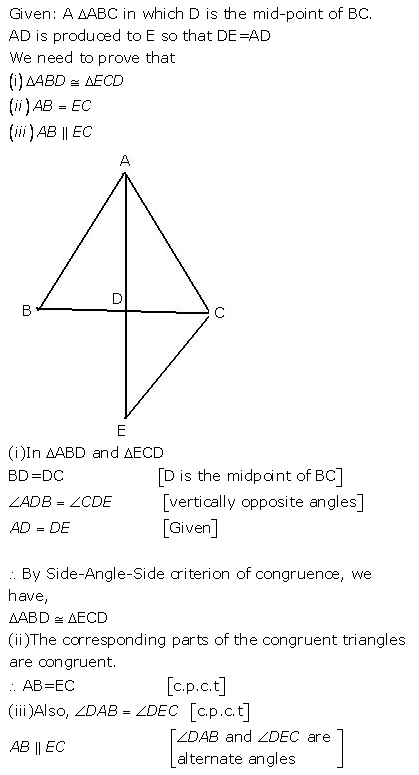Solution 5: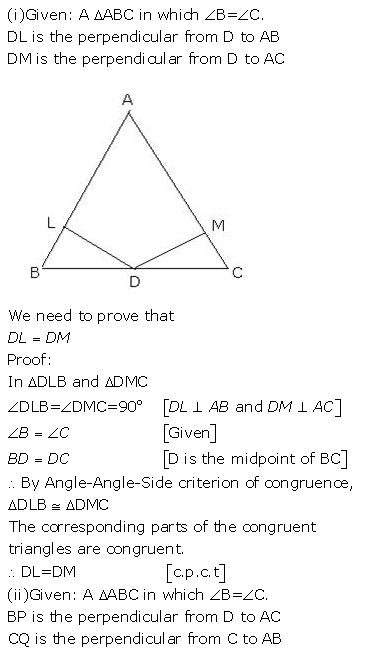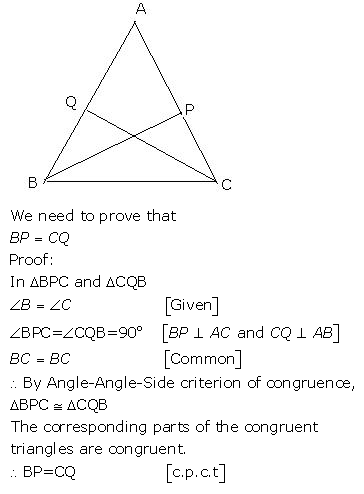Solution 6: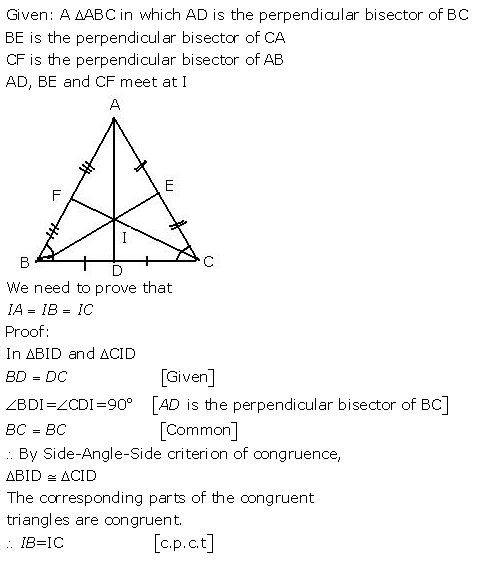Solution 7: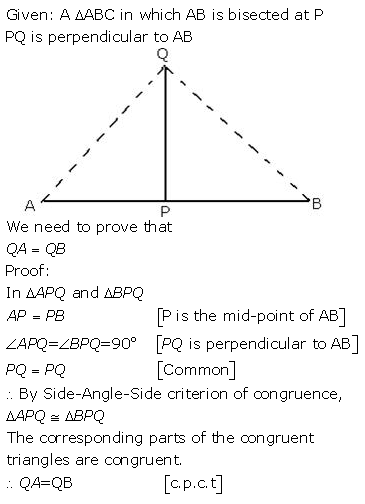Solution 8: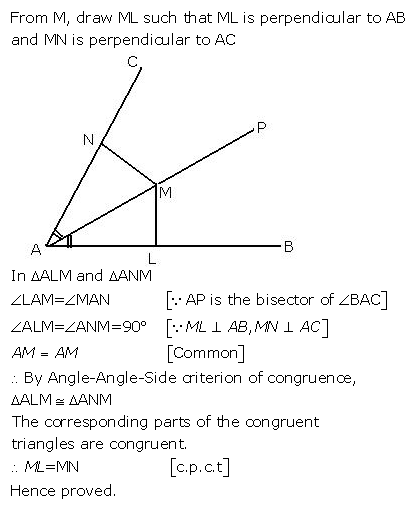Solution 9: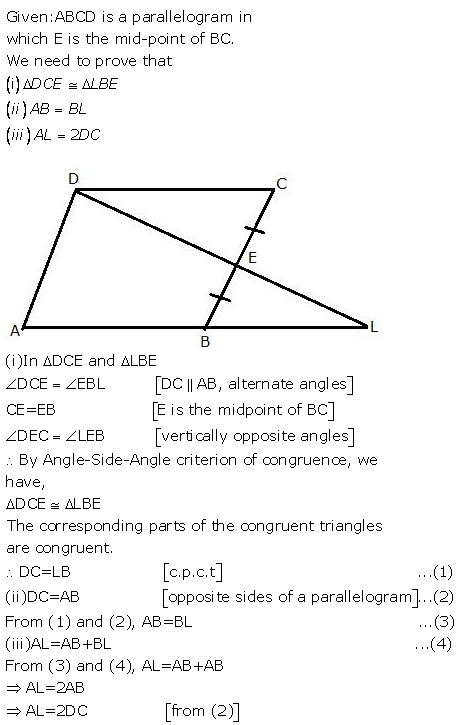Solution 10: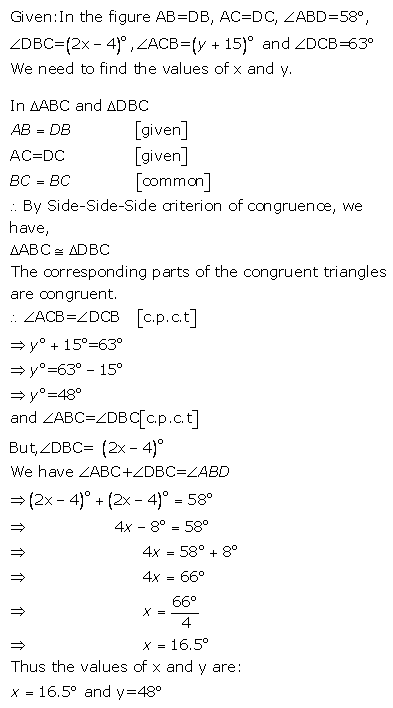Solution 11:Solution 12:Solution 13: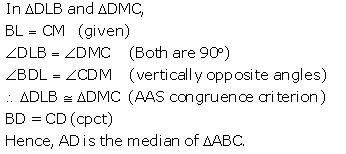Solution 14:Solution 15:Solution 16: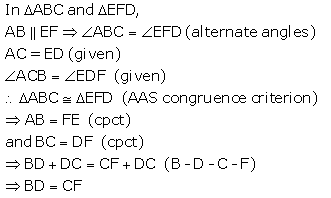Solution 17:Exercise 9(B)

Solution 1: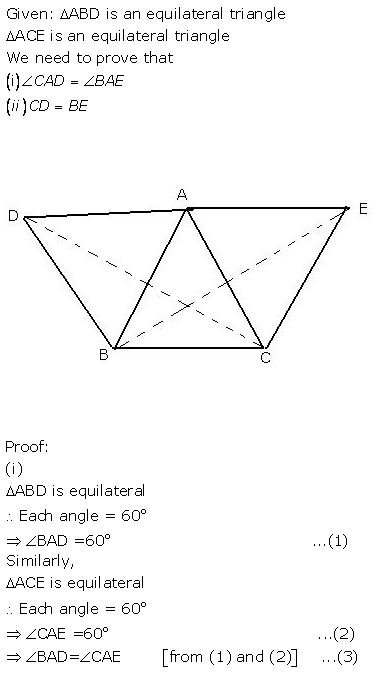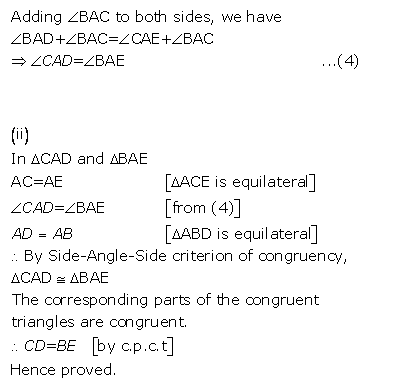Solution 2: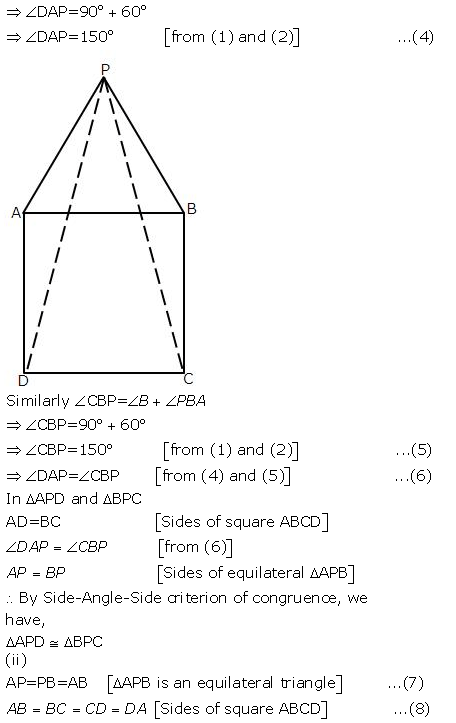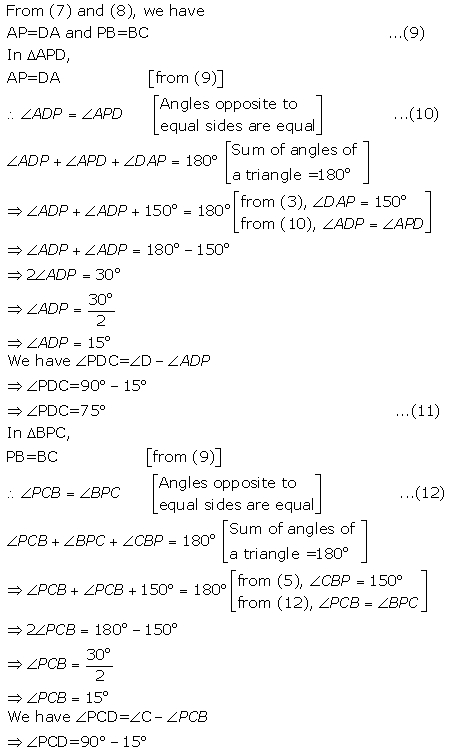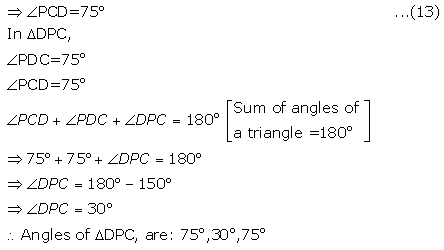Solution 3: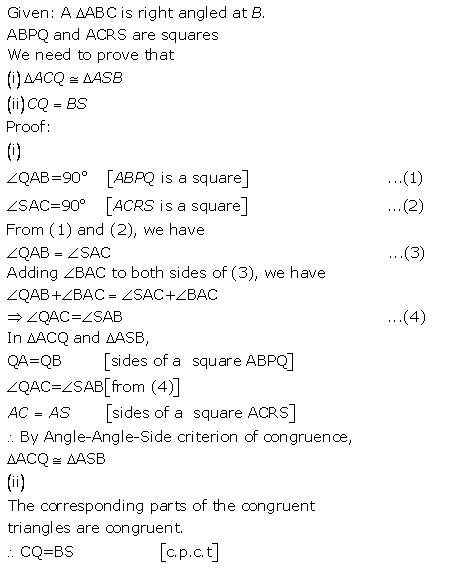Solution 4:Solution 5: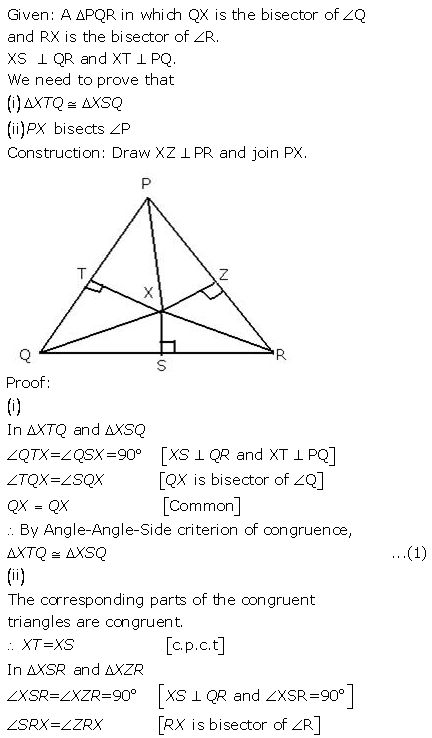Solution 6: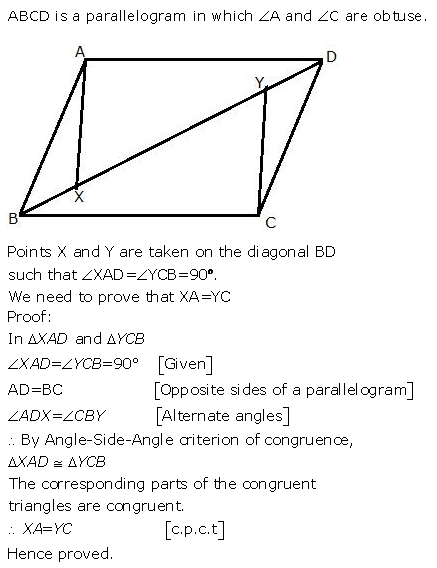Solution 7: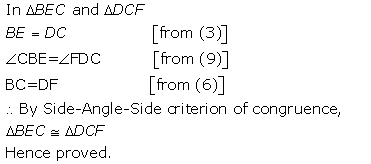Solution 8: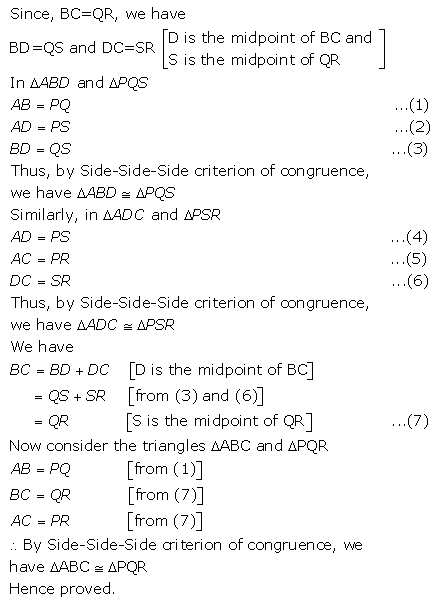Solution 9:Solution 10:Solution 11: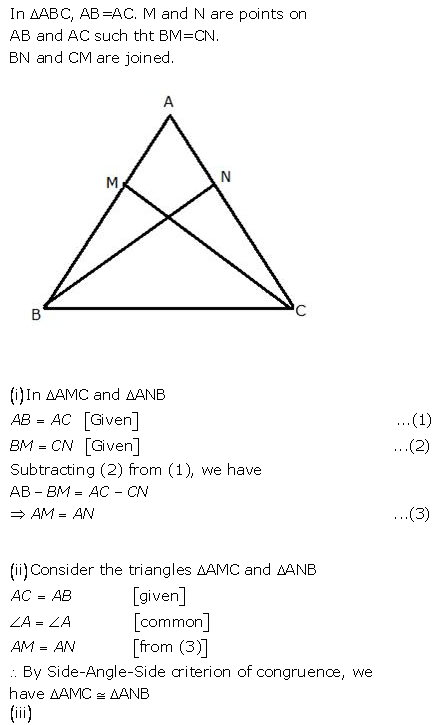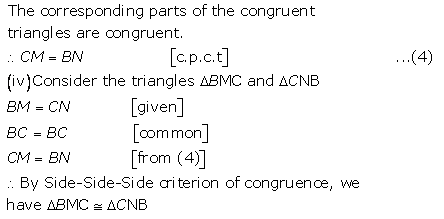Solution 12:Solution 13:Solution 14: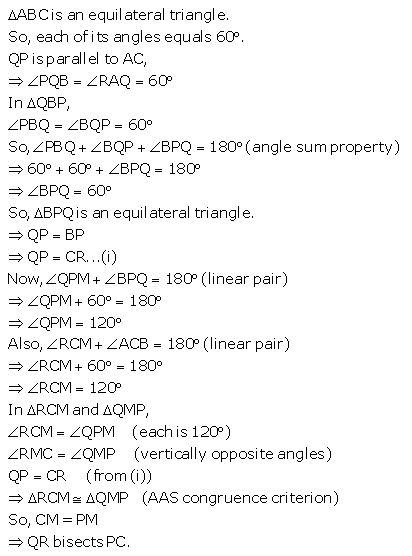Solution 15: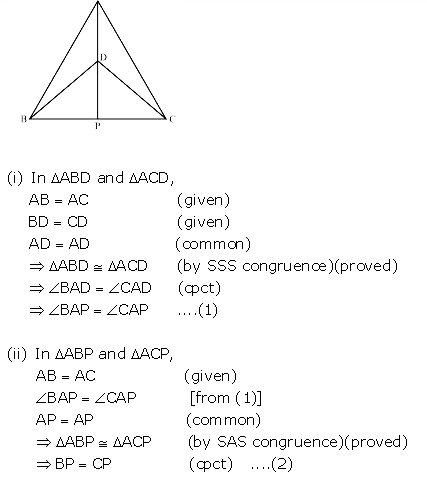Solution 16: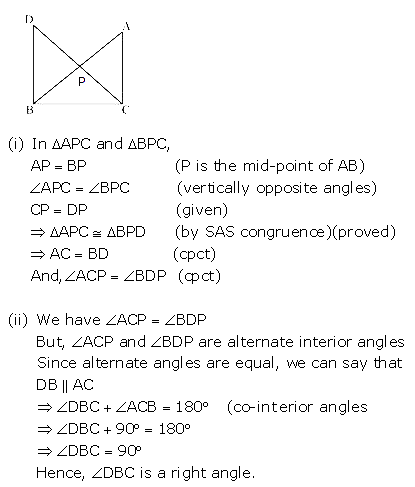Solution 17: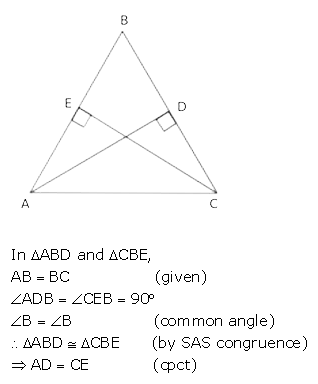Solution 18:Solution 19:Solution 20: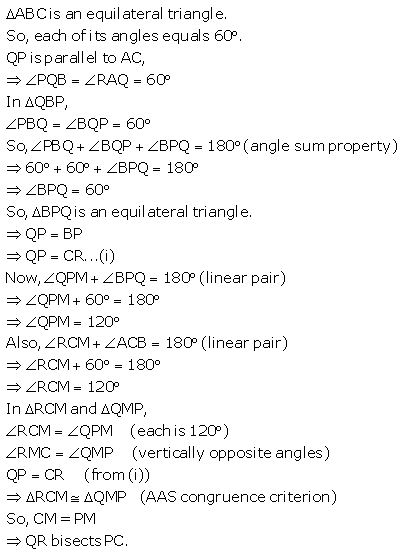More Resources for Selina Concise Class 9 ICSE Solutions